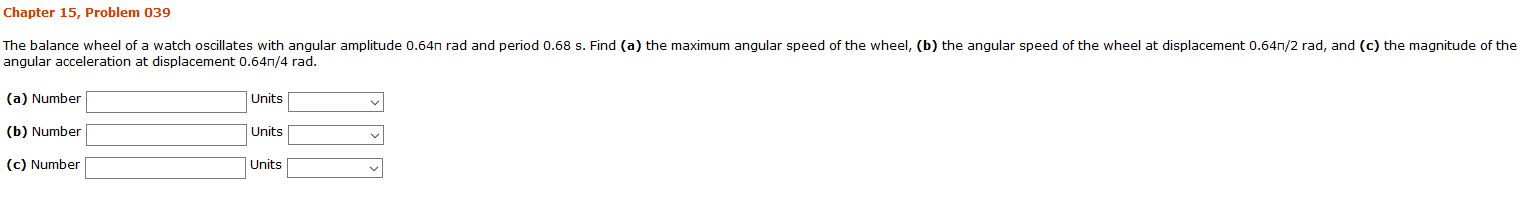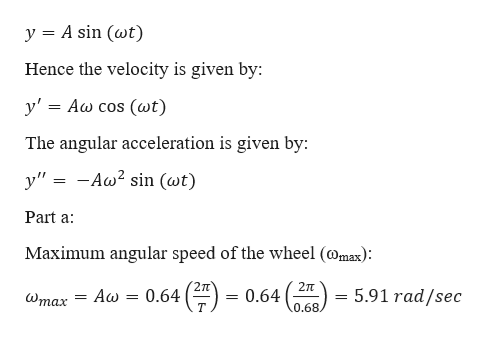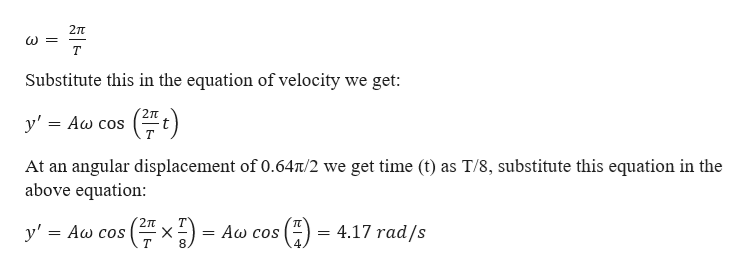# Chapter 15, Problem 039The balance wheel of a watch oscillates with angular amplitude 0.64n rad and period 0.68 s. Find (a) the maximum angular speed of the wheel, (b) the angular speed of the wheel at displacement 0.64n/2 rad, and (c) the magnitude of theangular acceleration at displacement 0.64n,/4 rad.(a) NumberUnits(b) NumberUnits(c) NumberUnits

Question
15 viewshelp_outlineImage TranscriptioncloseChapter 15, Problem 039 The balance wheel of a watch oscillates with angular amplitude 0.64n rad and period 0.68 s. Find (a) the maximum angular speed of the wheel, (b) the angular speed of the wheel at displacement 0.64n/2 rad, and (c) the magnitude of the angular acceleration at displacement 0.64n,/4 rad. (a) Number Units (b) Number Units (c) Number Units fullscreen
check_circle

Step 1

Given information:

Angular amplitude (A) = 0.64π rad

Time period (T) = 0.68 s

Step 2

The equation of motion for the oscillation is:help_outlineImage TranscriptioncloseA sin (wt) y Hence the velocity is given by: y' = Aw cos (wt) The angular acceleration is given by: y"-Aw2 sin (wt) Part a Maximum angular speed of the wheel (omax): 2T 2TT 0.64 = 0.64 5.91 rad/sec Aw Wmax = 0.68 fullscreen
Step 3

Part b:

We know ...help_outlineImage Transcriptionclose2T т Substitute this in the equation of velocity we get: y' Aw cos At an angular displacement of 0.64t/2 we get time (t) as T/8, substitute this equation in the above equation: xA cos 4.17 rad/s y' = Aw cos X = 4 fullscreen

### Want to see the full answer?

See Solution

#### Want to see this answer and more?

Solutions are written by subject experts who are available 24/7. Questions are typically answered within 1 hour.*

See Solution
*Response times may vary by subject and question.
Tagged in

### Angular Motion